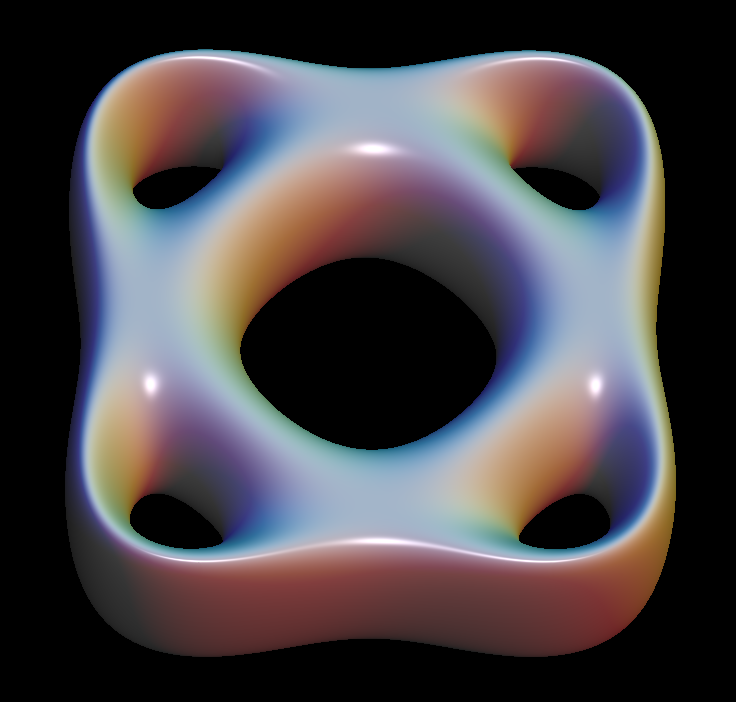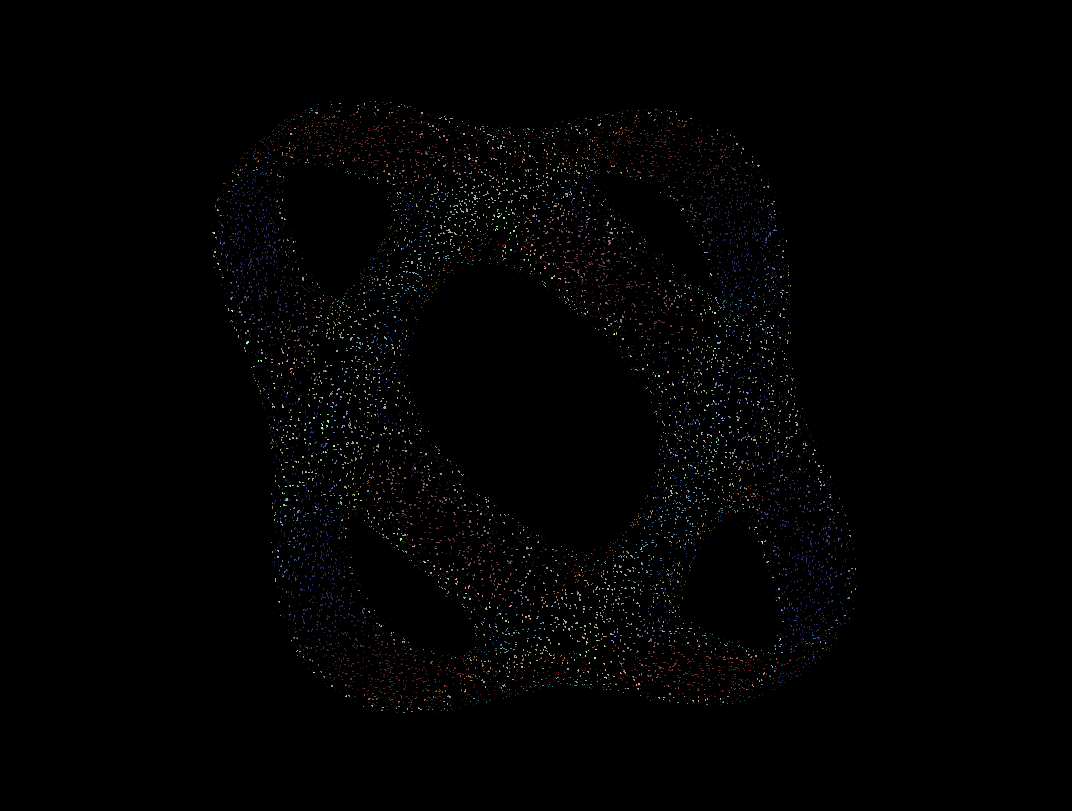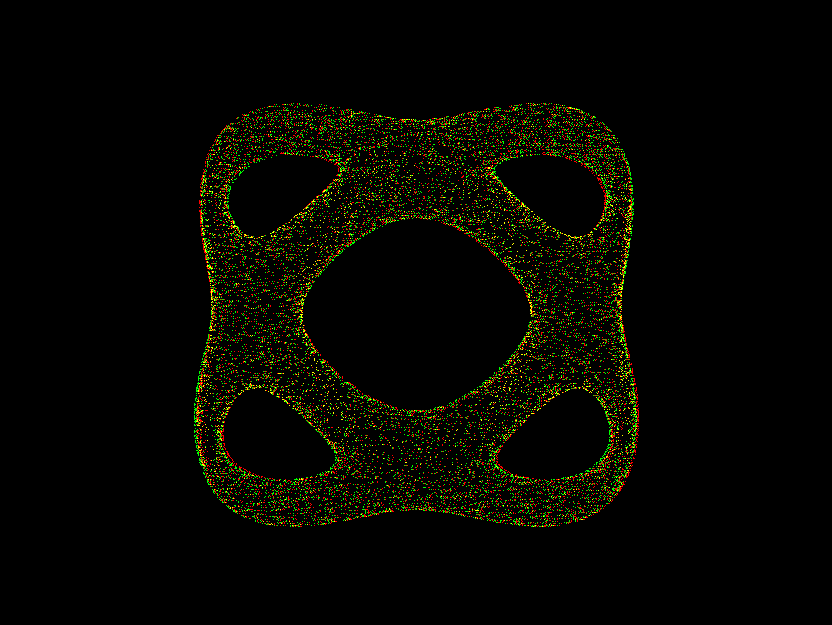# Bretzel5 Implicit Surface10 different levels of the Bretzel5 polynomial, p(x,y,z) = c for 10 different values of c.Bretzel5 001bretzel5 st
```the  Bretzel 5 surface the function f(x,y,z) is given by:
f(x,y,z) :=  (x^2 + (y^2)/4 - 1) * ( (x^2)/4 + y^2 - 1) - ff
(default: ff = 0.075)
```

Get red/blue stereo glasses from amazon

ImplicitCompact.pdf